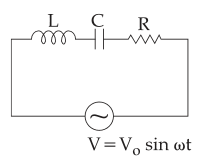Q

# Try this! For the LCR circuit, shown here, the current is observed to lead the applied voltage. An additional capacitor C', when joined with the capacitor C present in the circuit, makes the power factor of the circuit unity. The capacitor C'

For the LCR circuit, shown here, the current is observed to lead the applied voltage. An additional capacitor C', when joined with the capacitor C present in the circuit, makes the power factor of the circuit unity. The capacitor C', must have been connected in :• Option 1)

series with C and has a magnitude• Option 2)

series with C and has a magnitude• Option 3)

parallel with C and has a magnitude• Option 4)

parallel with C and has a magnitude666 Views

As we learnt in

Power factor -

Ratio of resistance and impedance.

-

After inserting  both become equal since

or

This implied  must reduced

This will happen when C is increased.

must be inserted in parellel to .

or  or

or

Option 1)

series with C and has a magnitudeThis is incorrect option.

Option 2)

series with C and has a magnitudeThis is incorrect option.

Option 3)

parallel with C and has a magnitudeThis is incorrect option.

Option 4)

parallel with C and has a magnitudeThis is correct option.

Exams
Articles
Questions